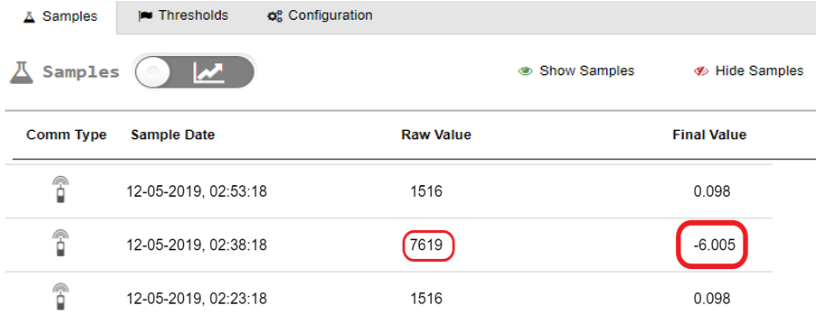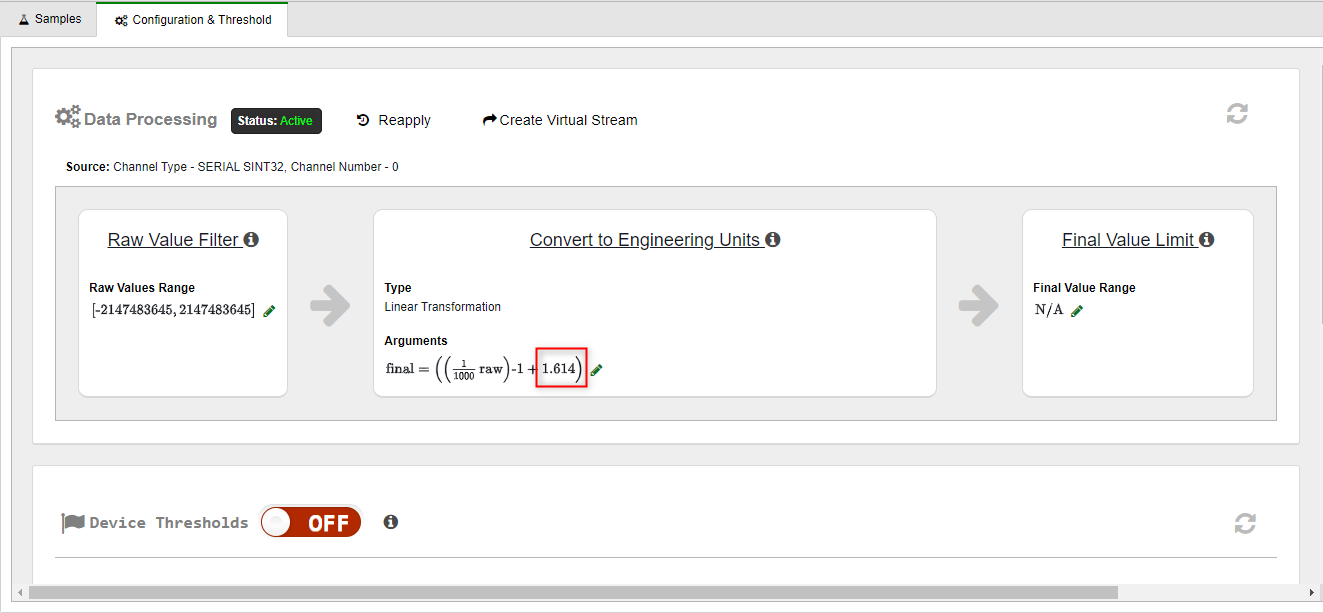# Correcting Negative Values from an Ultrasonic Level Sensor

## Problem: The Ultrasonic Level sensor is recording some negative values. Those values are found in the Final Value column in the Samples tab of a stream.

#### Why it's Happening

Negative values indicate bad readings by the sensor. If the sensor does not get a good reading, the value will be the full range of the sensor.

Factors such as rough water surface or some other type of interference can cause poor values.

#### Example

For this example, let's assume:

• Range of the sensor is about 7.6 meters
• The sensor is installed 1.614 meters from the bottom
• The sensor data values are in millimeters

The following table shows the sensor data in the Samples tab:Values in the Raw Value column indicate the distance from the Ultrasonic Level sensor face to the top of the water.

Values in the Final Value column are the result of converting the raw value to meters, and then  computing the distance from the Ultrasonic Level sensor face to the bottom of the water.

The Raw Value column shows a sensor reading of 7619, which is the sensor's range in millimeters. When that value is converted to engineering units, the value is -6.005 in the Final Value column.

The screenshot below shows the conversion to engineering units configuration in the Configuration & Threshold tab.The following calculations show the Convert to Eng. Units conversion:

`final = ((1/1000 raw)-1 + 1.614)      = ((7619/1000)-1  + 1.614)      = ((7.619)-1      + 1.614)      = (-7.619         + 1.614)      = -6.005`

#### Recommended Action

In the Configuration & Threshold tab, use Raw Value Filter to filter sensor data whose raw value is over the sensor's range in units of millimeters. Those values will not be used in charts and tables.

In the following screen shot, the default minimum is used. Set the maximum to be the range of your sensor. In this example, we set the maximum to 7000 for the Ultrasonic Level sensor.For details, see the following topics: